Function Repository Resource:

# TimeToFallFormula

Calculate the time to fall from a specified height

Contributed by: Julián Laverde
 ResourceFunction["TimeToFallFormula"][h] returns the time taken for an object to fall vertically from a distance h without drag. ResourceFunction["TimeToFallFormula"][h,assoc] returns the time taken for an object to fall vertically from a distance h with drag parameters in the Association assoc. ResourceFunction["TimeToFallFormula"][property] returns the specified property of the time to fall formula.

## Details and Options

The height h can be a number or a Quantity with length units. If given only as a number, the value is assumed to be in meters.
In ResourceFunction["TimeToFallFormula"][h, assoc], assoc can include the following basic elements:
 "DragCoefficient" 0.5 resistance of the object falling in the fluid "Area" 20 cm2 the object's projected area "Mass" 3 kg the object's mass "Density" 1.29 kg/m3 the fluid's density
The "DragCoefficient" can be a number greater than 0.
The object's projected "Area" can be a number or a Quantity with area units. If given only as a number, the value is assumed to be in square centimeters.
The object's "Mass" can be a number or a Quantity with mass units. If given only as a number, the value is assumed to be in kilograms.
The fluid's "Density" can be a number or a Quantity with density units. If given only as a number, the value is assumed to be in kilograms over cubic meters.
ResourceFunction["TimeToFallFormula"] can take the option "Gravity" to specify the gravitational acceleration "g". If unspecified, it is assumed to be equal to the "StandardAccelerationOfGravity".
Properties include:
 "FormulaWithDrag" equation for time to fall with drag "FormulaWithoutDrag" equation for time to fall without drag "QuantityVariableNames" English names for all variables

## Examples

### Basic Examples (2)

Calculate the time to fall without drag:

 In:=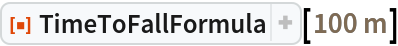Out=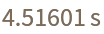Calculate the time to fall considering drag:

 In:=Out=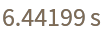### Scope (3)

Show the formula used with drag:

 In:=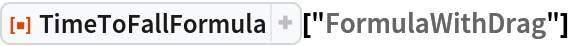Out=and without drag:

 In:=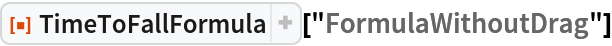Out=Find the name of the quantity variables used by TimeToFallFormula:

 In:=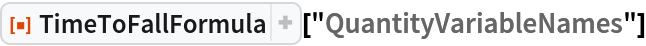Out=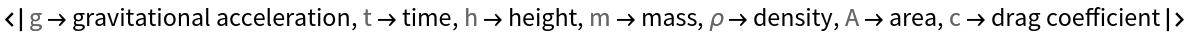TimeToFallFormula is listable:

 In:=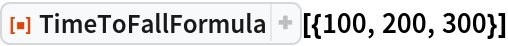Out=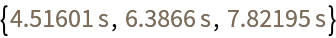### Options (1)

#### Gravity (1)

Use "Gravity" to specify the value of the gravity used:

 In:=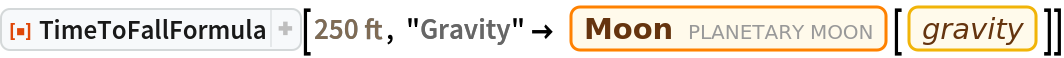Out=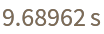### Properties and Relations (1)

Use Quantity to specify the units of the inputs:

 In:=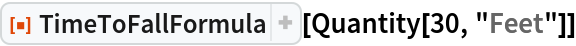Out=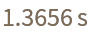### Neat Examples (2)

Plot the time that it would take to fall from the highest mountain of each continent:

 In:=Out=Plot the time that it would take to fall the height of the Empire State Building on each planet in the solar system:

 In:=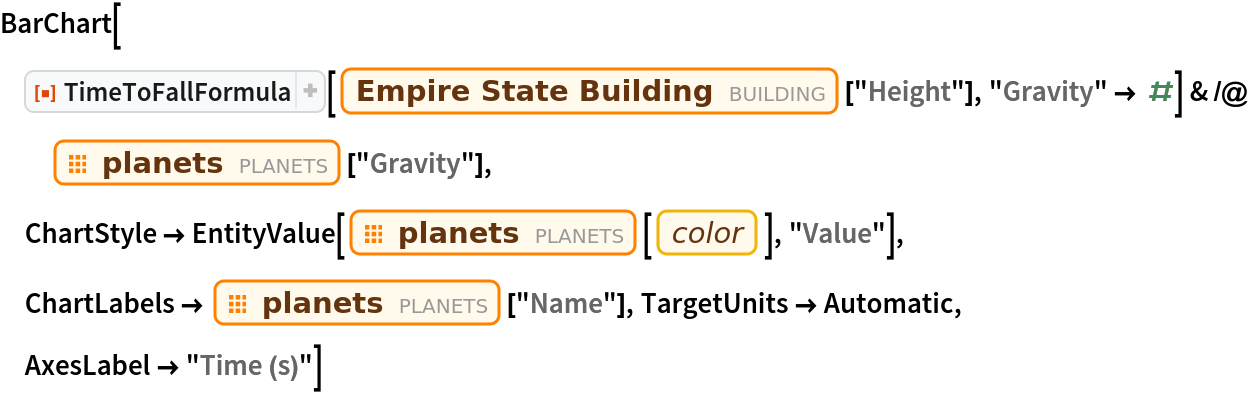Out=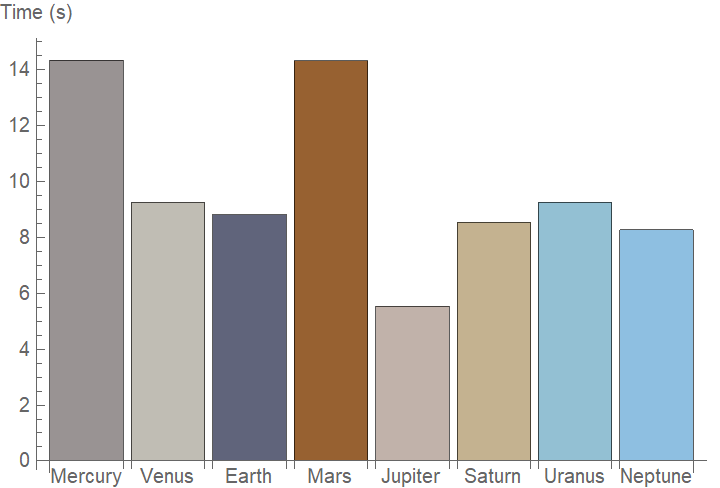## Publisher

WolframSpecialProjects

## Version History

• 1.0.0 – 01 March 2021